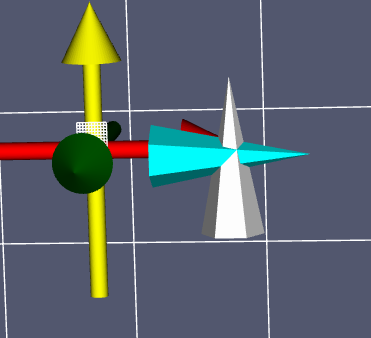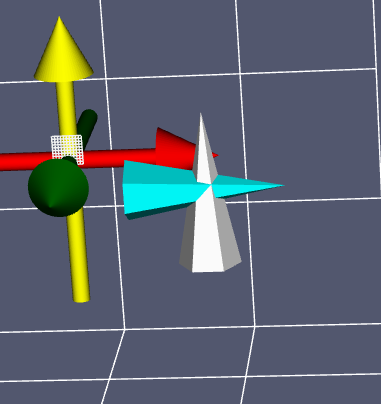# How to use rotateWXYZ

“Rotate the Prop3D in degrees about an arbitrary axis specified by
the last three arguments. The axis is specified in world
coordinates. To rotate an about its model axes, use RotateX,
RotateY, RotateZ.”

Questions are:

1. what is the origin of the rotation? We all know a rotation requires a rotation degree around a specific vector, and a vector has two points, how could the last three arguments of (x,y,z) define a rotation axis?
2. Does that implies the vector is defined by (x,y,z) and (0,0,0) in world coordinate? this is simple not true if you play around with it. it still rotate around the object origin.
3. does the (x,y,z) need to be normalized? In my view (0.3,0.3,0.3) and (1.3,1.3,1.3) is equivalent as to define the vector, but this is again not true.
4. if both rotateX and rotateWXYZ are rotations around the object origin, how could we rotate around a specific axis, for example, an axis defined by (-1,-1.-1) and (1,2,3)?

in the picture there are two identical cones placed in the same position to start with. Now I am rotating the grey cone with the following settings

actor.rotateWXYZ(90,0.3,0.3,0.3) ;

actor.rotateWXYZ(90,1.3,1.3,1.3) ;

actor.rotateWXYZ(90,0,0,1) ;actor.rotateZ(90) ;that last two pictures just prove my point that the rotateWXYZ rotation is a object local rotation, not a rotation in the world space.

rotateWXYZ(90,1.3,1.3,1.3) is not a rotation, it gives completely distorted object. why is that?

Could someone help to comment what is the supposed behavior of function rotateWXYZ(theta, x,y,z), thanks!

`rotateWXYZ(angleDegrees, x, y, z)` rotates an object in model/local space around an axis defined by the normalized vector (x,y,z). The origin of said axis is the center of the bounding box of the model.

The distortion likely is due to a non-normalized axis vector, resulting in scaling.

Thanks Forrest, that is clear and I’ll test it. I think it should replace the original text I quoted. Do you know the answer to the question 4? I mean, in reality, not all rotation is around the object center. Also, does rotateX, rotateY, rotateZ rotate around an prop3D’s center or its origin point?

As I checked Prop3D document, there is no function setCenter, but there is one setOrigin(x,y,z)
“Set the origin of the Prop3D. This is the point about which all rotations take place”

Everything works now. By applying setOrigin function I can make a rotation around any axis now.

1 Like